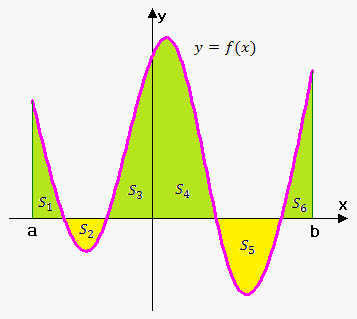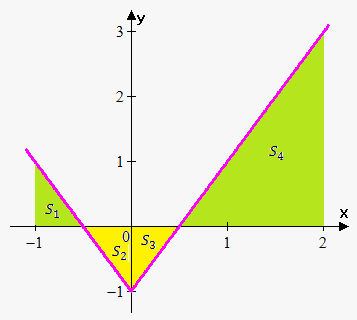# Concept of Definite Integral

## Related calculators: Definite and Improper Integral Calculator , Riemann Sum Calculator for a Function

In Area Problem note we saw that limit of the form lim_(n->oo)f(x_i^(**))Delta x arises when we compute an area.

It turns out that this same type of limit occurs in a wide variety of situations even when f is not necessarily a positive function.

Thus, this limit has special name and notation.

Definition of a Definite Integral. If f is a continuous function defined for a<=x<=b, we divide the interval [a,b] into n subintervals of equal width Delta x=(b-a)/n. We let x_0(=a),x_1,x_2,...,x_n(=b) be the endpoints of these subintervals and we choose sample points x_1^(**),x_2^(**),...,x_n^(**) in these subintervals, so x_i^(**) lies in the i-th subinterval [x_(i-1),x_i]. Then the definite integral of f from a to b is int_a^bf(x)dx=lim_(n->oo)sum_(i=1)^nf(x_i^(**))Delta x.

As with indefinite integral case f(x) is called integrand.

a is lower limit and b is upper limit.

Note, that definite integral is a number, it doesn't depend on x. We can choose another variable: int_a^bf(x)dx=int_a^bf(t)dt=int_a^bf(u)du.

The sum sum_(i=1)^nf(x_i^(**))Delta x is called Riemann sum.

If sample points x_i^** are left endpoints then sum is called Left Riemann Sum, if right endpoints then sum is called Right Riemann Sum.

Now let's see what will be if f can take both positive and negative values.If f takes on both positive and negative values, then the Riemann sum is the sum of the areas of the rectangles that lie above the x-axis minus sum of areas of the rectangles that lie below the x-axis.

Thus, definite integral is net area: area above x-axis minus area below x-axis:

int_a^bf(x)dx=S_1-S_2+S_3+S_4-S_5+S_6.

Note, that although we defined int_a^bf(x)dx by dividing interval [a,b] into n intervals of equal width, it is sometimes advantageous to work with intervals of unequal width. If the subinterval widths are Delta x_1,Delta x_2,...,Delta x_n then we have to ensure that all these widths approach 0 in the limiting process. This happens if the largest width, max Delta x_i , approaches 0. So in this case definition of integral becomes int_a^bf(x)dx=lim_(max Delta x_i->0) sum_(i=1)^nf(x_i^(**)) Delta x_i.

Now let's go through a couple of examples.

Example 1. Express lim_(n->oo)sum_(i=1)^n[3x_i^2-cos(x_i^5)]Delta x as an integral on the interval [0,pi] .

Comparing the given limit with the limit in definition of integral, we see that they will be identical if we choose f(x)=3x^2-cos(x^5) and x_i^(**)=x_i (so the sample points are right endpoints). We are given that a=0 and b=pi, therefore lim_(n->oo)sum_(i=1)^n[3x_i^2-cos(x_i^5)]Delta x=int_0^pi(3x^2-cos(x^5))dx.

It is very important to recognize limits of sums as integrals. In general, if lim_(n->oo)sum_(i=1)^nf(x_i^(**))Delta x=int_a^bf(x)dx then we replace lim sum by int, x_i^(**) by x and Delta x by dx.

Example 2. Evaluate int_0^2(3x^2-x^3)dx.

Divide interval [0,2] into n subintervals of width Delta x=(2-0)/n=2/n.

Since we can use any point within subinterval, let's use right endpoints.

Right endpoint of i-th subinterval is (2i)/n, so

int_0^2(3x^2-x^3)dx=lim_(n->oo)sum_(i=1)^nf((i)/n)2/n=lim_(n->oo)sum_(i=1)^n (3((2i)/n)^2-((2i)/n)^3)2/n=lim_(n->oo)sum_(i=1)^n(24 i^2/n^2-16i^3/n^3)1/n=

=lim_(n->oo)sum_(i=1)^n(24/n^3 i^2-16/n^4 i^3)=lim_(n->oo)(sum_(i=1)^n(24/n^3 i^2)-sum_(i=1)^n(16/n^4 i^3))=lim_(n->oo)(24/n^3 sum_(i=1)^n i^2-16/n^4 sum_(i=1)^n i^3)=

On this stage we need the following two formulas:

sum_(i=1)^n i^2=(n(n+1)(2n+1))/6.

sum_(i=1)^ni^3=((n(n+1))/2)^2.

So, int_0^2(3x^2-x^3)dx=lim_(n->oo)(24/n^3 (n(n+1)(2n+1))/6-16/n^4 ((n(n+1))/2)^2)=

=lim_(n->oo)((4(n+1)(2n+1))/n^2-(4(n+1)^2)/n^2)=

=lim_(n->oo)(4(1+1/n)(2+1/n)-4(1+2/n+1/n^2))=4(1+0)(2+0)-4(1+2*0+0)=4

So, int_0^2(3x^2-x^3)dx=4.

Example 3. Evaluate the following integral by interpreting it in terms of areas: int_(-2)^2sqrt(4-x^2)dx.

Since f(x)=sqrt(4-x^2)>=0, we can interpret this integral as the area under the curve y=sqrt(4-x^2) from -2 to 2.

Squaring both sides give y^2=4-x^2 then x^2+y^2=4 and the required area is area of semicircle with radius 2.

Therefore, int_(-2)^2sqrt(4-x^2)dx=1/2 pi*(2)^2=2pi .

Example 4. Evaluate the following integral by interpreting it in terms of areas: int_-1^2(2|x|-1)dx.First we draw graph of the function y=2|x|-1 on interval [-1,2].

Recall that definite integral is net area: int_(-1)^2(2|x|-1)dx=S_1-S_2-S_3+S_4.

Now area S_1 is area of right-angled triangle with legs 1/2 and 1, so S_1=1/2*1/2*1=1/4.

Similarly, S_2=1/2*1/2*1=1/4, S_3=1/2*1/2*1=1/4 and S_4=1/2*3/2*3=9/4.

So, int_(-1)^2 (2|x|-1)dx=1/4-1/4-1/4+9/4=2.